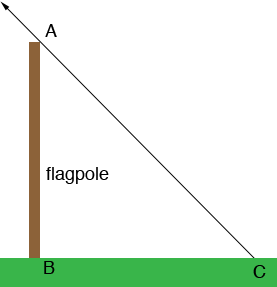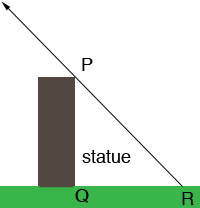SEARCH HOMEMath Central Quandaries & QueriesQuestion from Krista, a student: A flagpole casts a ten meter (m) shadow at the same time as a six metre (m) statute beside it casts a two metre shadow. What is the height of the flagpole??We have two responses for you

Hi Krista,

If you have two objects casting shadows on level ground at the same time then the sun makes the same angle at the tip of the shadow.Thus angles BCA and PQR are congruent. Both triangles ABC and PQR are right triangles and since two of their angles are congruent the triangles are similar.

Use what you know about similar triangles.

If yo need further assistance write back.

Penny

Hi Krista.

These are similar right triangles. In this situation, the ratios of
corresponding sides are equal.

Let x = the height of the flagpole.

Then x / 6 = 10 / 2. Solve for x.

Cheers,
Stephen La Rocque.Math Central is supported by the University of Regina and The Pacific Institute for the Mathematical Sciences.